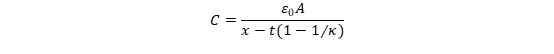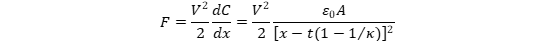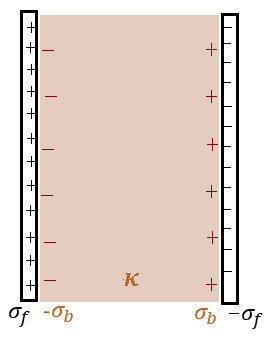# Formula for force between two sheets of aluminum foil under voltage

• A
andymag
Hi guys,

I am struggling to find the answer on Google and at forums. I am experimenting with two sheets of aluminum foil that are separated by a thin plastic foil. In theorie they should be attracted to each other if I connect one foil to power and other to ground of a DC voltage source. I am aware that I probably need very high voltages to achieve a visible effect. However, I would love to know the formula to be able to calculate the attraction force between the two sheets. Does anyone know an approach?

Thank you very much in advance
Bests
Andy

Gold Member
It would probably have something to do with electrostatics. Effectively what you're making is a capacitor, with a plastic foil for the dielectric.
I'm not sure off the top of my head what it would be, but you could probably treat each foil sheet as having a constant charge density ##\rho## (one positive the other negative), then integrating the electrostatic force across the sheet. That should at least get you something reasonable to start with for comparison with your experiments, and would give you an idea of the magnitude of the force.
Try looking into capacitance.
Edit:
It seems like you don't even need to do all that.
https://physics.stackexchange.com/q...e-of-attraction-between-plates-of-a-capacitor

Gold Member
2022 Award
Look for the Maxwell tensor for the electromagnetic field.

Another back-of-the-envelope estimate is for capacitors with large plates and small distance between the plates as follows: You can then assume that there's a homogeneous electric field between the plates and neglect the field outside. Then the total energy is
$$\mathcal{E}=\int_V \mathrm{d}^3 x \frac{1}{2} \vec{E} \cdot \vec{D}=\frac{V \epsilon_0 \epsilon_r}{2} E^2 =\frac{\epsilon_0 \epsilon_r Q^2}{2d}.$$
If you keep the charge ##Q## constant then
$$F=-\partial_d \mathcal{E}=\frac{\epsilon_0 \epsilon_r Q^2}{2d^2}.$$
You can think about what changes, when you keep the voltage across the capacitor constant.

andymag
Hi BiGyElLoWhAt and vanhees71,

that was a huge help from both of you. Thank you very much! I understood the formulas. However, I cannot get into my head that the thickness and material (e.g., copper vs alu) of the plates in a parallel plate capacitor do not matter. My logic is that if the plates are thicker then more electrons can be pushed into or drawn from the plate.

This is probably a very easy question for you but I would appreciate if you could help me understand.

Andy

SDL
Let's perform some calculations. We have a coductive plate of size 10x10cm and 1mm thick. Its volume is ##(10^{-1})^2 \cdot 10^{-3}=10^{-5}~\rm{m^3}##. Taking the "typical" size of an atom of ##0.1~\rm{nm}=10^{-10}~\rm{m}## hence its volume ##10^{-30}~\rm{m^3}##, the plate contains ##10^{-5} / 10^{-30} = 10^{25}## atoms. To simplify our silution, assume that every atom has only one free electron in its outermost shell, taking part in conductivity. Then, if we take away only one electron from each atom, the total charge the plate can have is ##e \cdot 10^{25}=1.6 \cdot 10^{-19}~\rm{C} \cdot 10^{25}=1.6 \cdot 10^{6}~\rm{C}##.
This charge is so huge, that it's hard to imagine! Calculations show that a conductive body of "common" dimensions, even very thin, would always have enough free electrons to give it any charge needed in practice.

andymag
Hi SDL,

thanks a lot! If I understand correctly, the thickness of the plates does not matter because there is no practical way to charge a parallel plate capacitor even remotely close to its true ability to contain excess electrons, which makes kinda sense since the more electrons are in a plate the more difficult (more power needed) it becomes to add additional ones. Now I understand why we need such a relatively huge voltage to achieve relatively "low" charges. Or are these thoughts wrong?

But what about graphene? Suppose, we could build two parallel capacitor plates consisting only of one atom layer of graphene. Would it also be enough? 1.6 * 10^6 C / 10^13 = 1.6 * 10^(-7) = 100 nC?

Thank you very much in advance!
Cheers
Andy

Dilwyn Jones
I just did some work on this for a client. With part film, part air in the gap (eg Duffin - Electricity and Magnetism):
The system could be modelled as a parallel plate capacitor with the gap x between conducting surfaces, area A, partly filled by dielectric, thickness t and dielectric constant k. The capacitance C is given by:And the attractive force between the plates held at a potential difference V:This assumes the plates remain perfectly parallel and they are large compared with the gap.
Hope this helps.

Mentor
thanks a lot! If I understand correctly, the thickness of the plates does not matter because there is no practical way to charge a parallel plate capacitor even remotely close to its true ability to contain excess electrons, which makes kinda sense since the more electrons are in a plate the more difficult (more power needed) it becomes to add additional ones. Now I understand why we need such a relatively huge voltage to achieve relatively "low" charges. Or are these thoughts wrong?
The limiting factor in a parallel plate capacitor arrangement is the arc-through Electric field level. If you charge the capacitor up to too high of a voltage, you generate an arc through the dielectric (or vacuum) between the plates. Different dielectric materials have different arc-through E-field values, and low-pressure gas and vacuum arc-through E-field values are described by the Paschen Curves:

https://en.wikipedia.org/wiki/Paschen's_law

To calculate the E-field value for different dielectrics and different capacitor plate spacings, you would use the basic capacitor equations (which assume as mentioned above that the plate spacing d is much less than the plate dimensions for the area A):
$$Q=CV$$
$$C=\epsilon \frac{A}{d}$$
$$E = \frac{V}{d}$$
Where ##\epsilon = \epsilon_0 \epsilon_r## which takes into account the relative permittivity of the dielectric. I haven't worked through the equations to figure out how to get the maximum force (what is the optimum dielectric and spacing, given the constraints imposed by arc-through issues), but you might try using these equations and the previous force equations to work through that...Last edited:
Homework Helper
Gold Member
Assume the dielectric fills the space between the parallel conducting plates:Neglecting edge effects, the force on the left sheet is due just to the electric field produced by the free charge ##-\sigma_f## on the right sheet. The bound charges ##\sigma_b## and ##-\sigma_b## produce no net force on the left sheet. The magnitude of the field produced by the free charge of the right sheet is ##\large \frac{\sigma_f}{2\varepsilon_0}##. Thus, the force on the left sheet is $$F = Q \left(\frac{\sigma_f}{2 \varepsilon_0} \right)= \frac{Q^2}{2 \varepsilon_0 A}.$$ Here, ##A## is the area of a sheet and ##Q## is the free charge stored on a sheet. ##Q## is related to the potential difference between the plates as ##Q = CV = \large \frac{\varepsilon_0 \kappa A}{d} V## where ##d## is the distance between the plates and ##\kappa## is the dielectric constant. So, the force on the left plate is $$F = \frac{\varepsilon_0 A}{2}\left(\frac{\kappa V}{d}\right)^2$$ This agrees with @Dilwyn Jones's equation for the case where the dielectric fills the space between the plates.

The weight of a plate is ##\rho A t g## where ##\rho## is the mass density of the material of the plate, ##t## is the thickness of the plate, and ##g## is the acceleration due to gravity. So, the ratio ##R## of the electric force on a plate to its weight is $$R = \frac{\varepsilon_0}{2 \rho g t} \left(\frac{\kappa V}{d}\right)^2.$$ Thus, the voltage required to make the electric force match the weight of a plate is $$V = \sqrt{\frac{2\rho g t}{\varepsilon_0}}\frac{d}{\kappa}.$$
As an example, let the plates be thin sheets of aluminum foil of thickness ##t = 0.1## mm and mass density ##\rho = 2.7## g/cm3. Let the dielectric be polystyrene (##\kappa = 2.6##) and the distance between the plates be ##d = 2 ##mm. The voltage required to make the electric force equal the weight of a foil sheet is then about 600 V. This makes the electric field inside the polystyrene equal to about ##3 \times 10^5## V/m which is well below the dielectric strength of polystyrene (approx. ##2 \times 10^7## V/m).

•berkeman
SDL
there is no practical way to charge a parallel plate capacitor even remotely close to its true ability to contain excess electrons
Yes, this is exactly what I intended to show.
the more electrons are in a plate the more difficult (more power needed)
Almost true except "voltage" instead of "power", which is a significantly different quantity.
relatively huge voltage to achieve relatively "low" charges. Or are these thoughts wrong?
This is what capacitance is for: it depends on capacitor geometry, dielectric used, technology and not on electrical parameters like voltage and current (in linear systems). And it defines the "ability" of the capacitor to accept charge: since ##C=\frac Q V##, the greater the charge pumped up and the less the voltage required, the greater the capacitance.

Dilwyn Jones
All good stuff. Just pointing out:
The electrons in the conductors are strongly attracted towards or repelled away from the inner surface, so there is is zero field within the bulk of the plates. As the voltage is raised, electrons start escaping through breakdown of the air or dielectric, or by field emission in a vacuum, long before all the states in the conduction band are filled.
An air gap reduces the attractive force between the plates, if the metal to dielectric contact is not intimate.
If free charge gets onto the dielectric surface, the force is reduced (not accounted for in the equations).